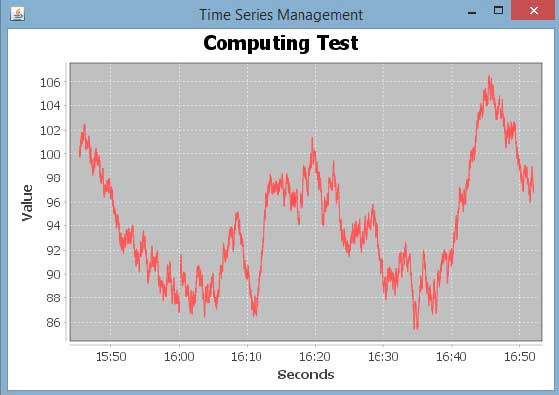# JFreeChart - TimeSeries Chart

A time series chart displays sequence of data points, which varies at equal intervals of time. This chapter demonstrates — how we can use JFreeChart to create Time Series Chart from a given set of business data.

Let us consider various random numbers generated by using standard Java API Math.random(). We use these numbers to generate a Time Series Chart. You can generate similar chart for total number of errors occurring in your website at a given interval of time.

## AWT Based Application

Following is the code to create Time Series Chart from the numbers generated by Math.random() at a given time internal.

```import org.jfree.chart.ChartFactory;
import org.jfree.chart.ChartPanel;
import org.jfree.chart.JFreeChart;
import org.jfree.data.general.SeriesException;
import org.jfree.data.time.Second;
import org.jfree.data.time.TimeSeries;
import org.jfree.data.time.TimeSeriesCollection;
import org.jfree.data.xy.XYDataset;
import org.jfree.ui.ApplicationFrame;
import org.jfree.ui.RefineryUtilities;

public class TimeSeries_AWT extends ApplicationFrame {

public TimeSeries_AWT( final String title ) {
super( title );
final XYDataset dataset = createDataset( );
final JFreeChart chart = createChart( dataset );
final ChartPanel chartPanel = new ChartPanel( chart );
chartPanel.setPreferredSize( new java.awt.Dimension( 560 , 370 ) );
chartPanel.setMouseZoomable( true , false );
setContentPane( chartPanel );
}

private XYDataset createDataset( ) {
final TimeSeries series = new TimeSeries( "Random Data" );
Second current = new Second( );
double value = 100.0;

for (int i = 0; i < 4000; i++) {

try {
value = value + Math.random( ) - 0.5;
series.add(current, new Double( value ) );
current = ( Second ) current.next( );
} catch ( SeriesException e ) {
}
}

return new TimeSeriesCollection(series);
}

private JFreeChart createChart( final XYDataset dataset ) {
return ChartFactory.createTimeSeriesChart(
"Computing Test",
"Seconds",
"Value",
dataset,
false,
false,
false);
}

public static void main( final String[ ] args ) {
final String title = "Time Series Management";
final TimeSeries_AWT demo = new TimeSeries_AWT( title );
demo.pack( );
RefineryUtilities.positionFrameRandomly( demo );
demo.setVisible( true );
}
}
```

Let us keep the above Java code in TimeSeries_AWT.java file, and then compile and run it from the command prompted as −

```\$javac TimeSeries_AWT.java
\$java TImeSeries_AWT
```

If everything is fine, it will compile and run to generate the following Time Series Graph −## JPEG Image Creation

Let us re-write the above example to generate a JPEG image from a command line.

```import java.io.*;

import org.jfree.chart.ChartFactory;
import org.jfree.chart.JFreeChart;
import org.jfree.data.general.SeriesException;
import org.jfree.data.time.Second;
import org.jfree.data.time.TimeSeries;
import org.jfree.data.time.TimeSeriesCollection;
import org.jfree.data.xy.XYDataset;
import org.jfree.chart.ChartUtilities;

public class TimeSeriesChart {

public static void main( final String[ ] args )throws Exception {
final TimeSeries series = new TimeSeries( "Random Data" );
Second current = new Second();
double value = 100.0;

for ( int i = 0 ; i < 4000 ; i++ ) {

try {
value = value + Math.random( ) - 0.5;
series.add( current , new Double( value ) );
current = ( Second ) current.next( );
} catch ( SeriesException e ) {
System.err.println( "Error adding to series" );
}
}

final XYDataset dataset=( XYDataset )new TimeSeriesCollection(series);
JFreeChart timechart = ChartFactory.createTimeSeriesChart(
"Computing Test",
"Seconds",
"Value",
dataset,
false,
false,
false);

int width = 560;   /* Width of the image */
int height = 370;  /* Height of the image */
File timeChart = new File( "TimeChart.jpeg" );
ChartUtilities.saveChartAsJPEG( timeChart, timechart, width, height );
}
}
```

Let us keep the above Java code in TimeSeriesChart.java file, and then compile and run it from the command prompted as −

```\$javac TimeSeriesChart.java
\$java TimeSeriesChart
```

If everything is fine with your environment, it will compile and run to create a JPEG image file TimeChart.jpeg file in your current directory.